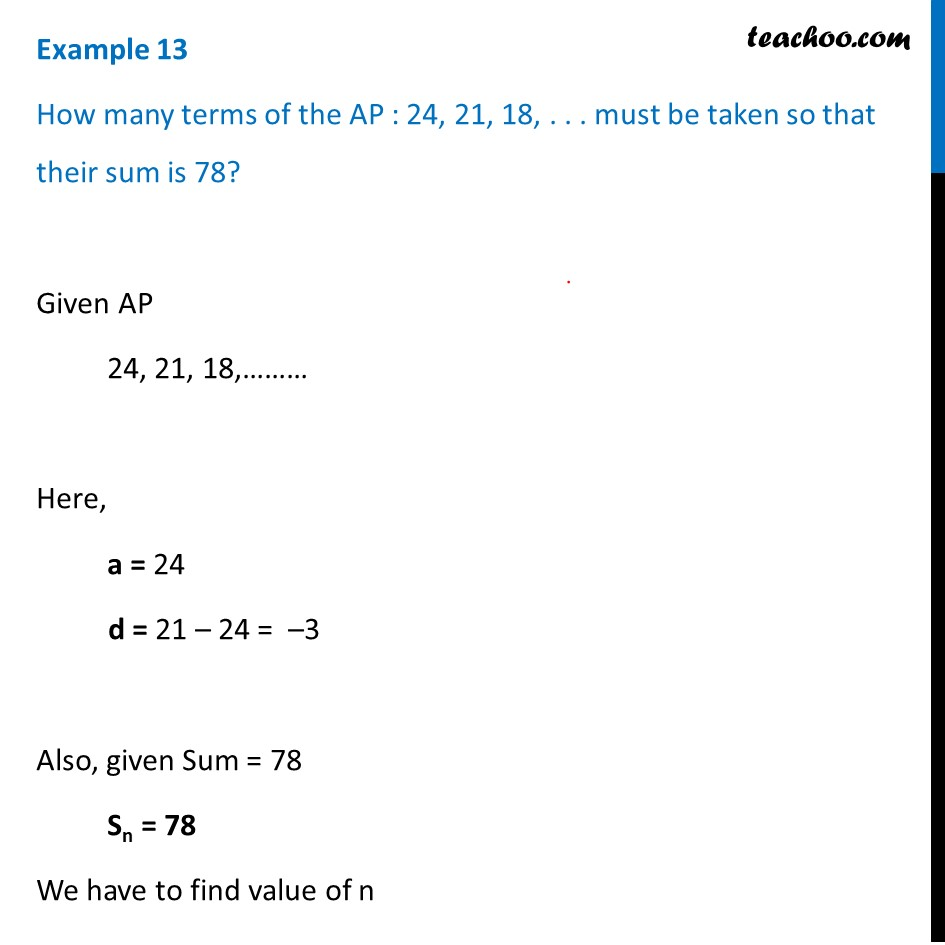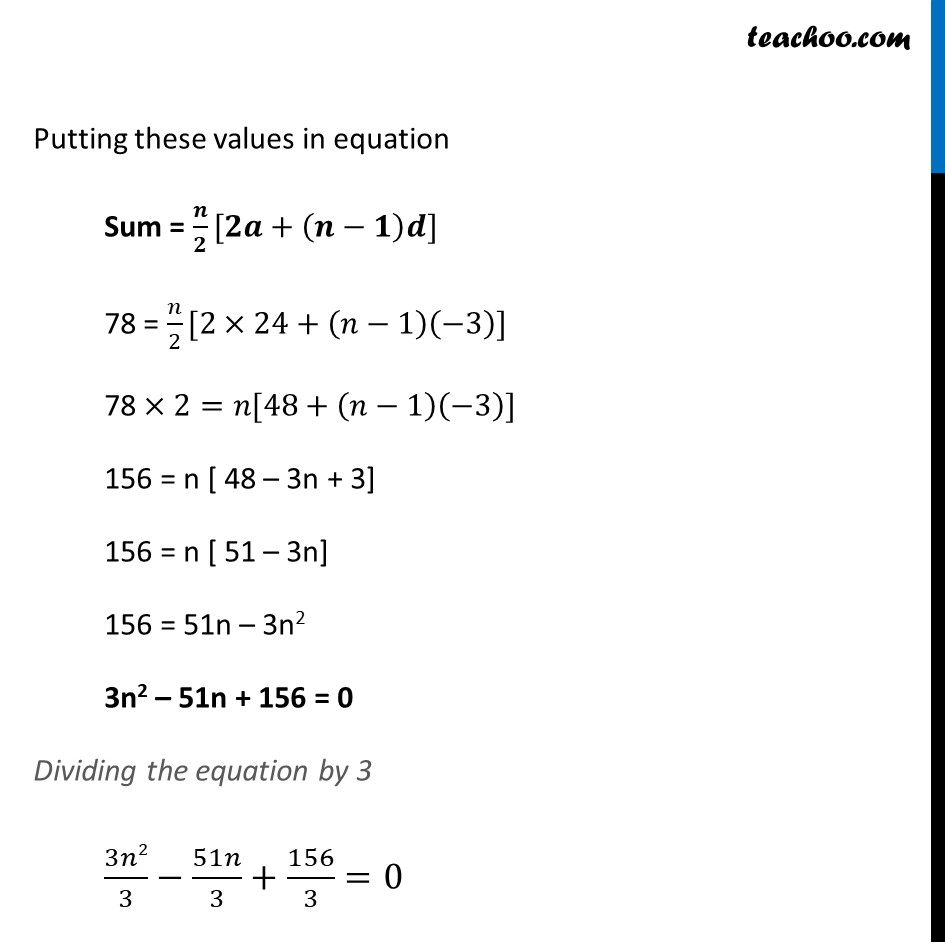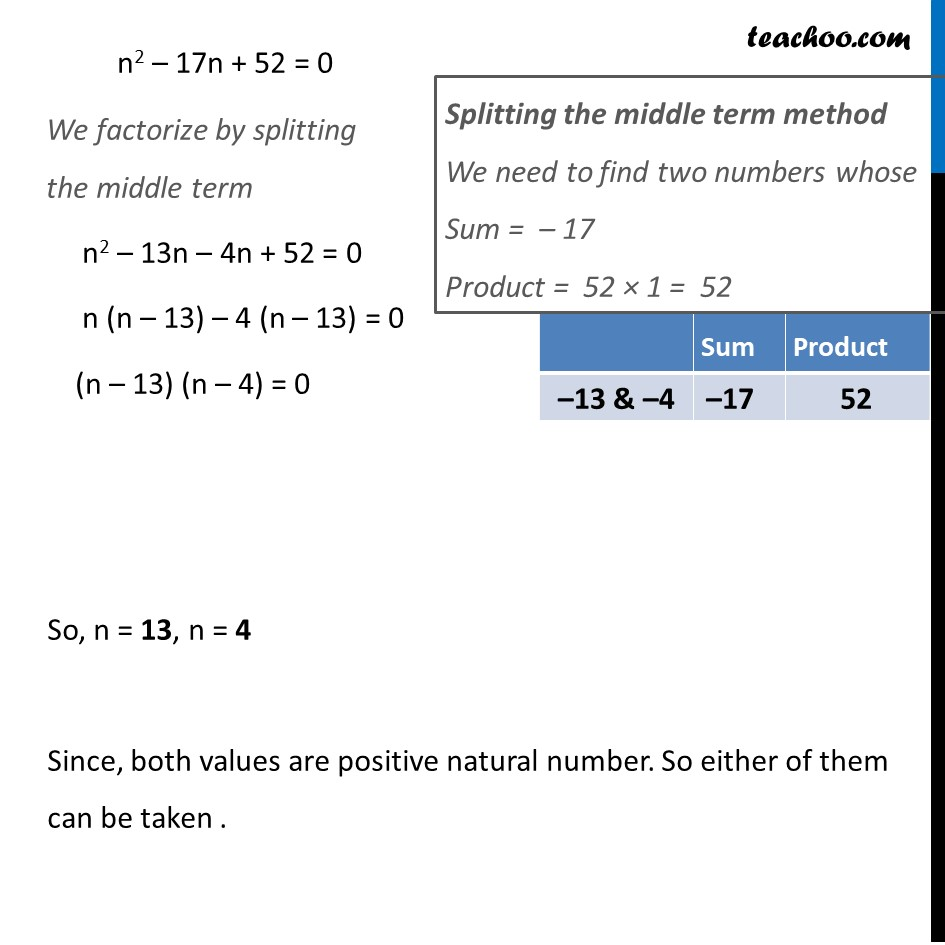Examples

Chapter 5 Class 10 Arithmetic Progressions
Serial order wiseLearn in your speed, with individual attention - Teachoo Maths 1-on-1 Class

### Transcript

Example 13 How many terms of the AP : 24, 21, 18, . . . must be taken so that their sum is 78? Given AP 24, 21, 18,……… Here, a = 24 d = 21 – 24 = –3 Also, given Sum = 78 Sn = 78 We have to find value of n Putting these values in equation Sum = 𝒏/𝟐[𝟐𝒂+(𝒏−𝟏)𝒅] 78 = 𝑛/2[2×24+(𝑛−1)(−3)] 78 ×2=𝑛[48+(𝑛−1)(−3)] 156 = n [ 48 – 3n + 3] 156 = n [ 51 – 3n] 156 = 51n – 3n2 3n2 – 51n + 156 = 0 Dividing the equation by 3 3𝑛2/3−51𝑛/3+156/3=0 n2 – 17n + 52 = 0 We factorize by splitting the middle term n2 – 13n – 4n + 52 = 0 n (n – 13) – 4 (n – 13) = 0 (n – 13) (n – 4) = 0 Splitting the middle term method We need to find two numbers whose Sum = – 17 Product = 52 × 1 = 52 So, n = 13, n = 4 Since, both values are positive natural number. So either of them can be taken .